# Gray code

(diff) ← Older revision | Latest revision (diff) | Newer revision → (diff)
Jump to: navigation, search

Let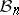denote the set of all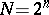binary strings of length. A Gray code of orderis a sequenceof elements ofin which every successive pair () of bitstrings differs by one bit in some position; the bits in all other positions agree. A cyclic Gray code is one in whichand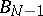also differ by one bit. These bitstrings can also be thought of as the characteristic vectors of subsets of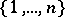. The hypercube of order(or-cube) is the graph whose vertex set isand whose edge set consists of those pairs of vertices that differ by one bit. Thus, a Gray code is a Hamilton path (cf. Graph circuit) on the-cube and a cyclic Gray code is a Hamilton cycle (cf. Graph circuit) on the-cube.

### Origins and uses.

The term "Gray code" comes from F. Gray, who worked as an engineer at Bell Laboratories, and who, in 1953, obtained US patent 2,632,058 for "pulse code communication" . The patent contained a construction which has come be known as the binary reflected Gray code (BRG code), after the recursive construction rule given below for.(a1)

If, then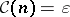, the empty string. There are three operations. The dot operation, for a list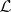, gives the list consisting of every string inappended with the symbol. The concatenation operation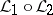gives the list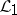followed by the list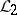. By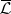one denotes the reversal of the list. Thus,and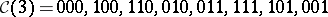.

There is a simple relation between theth binary string (counting from) in the BRG code, call it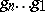, and the binary representation of. For,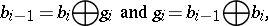where the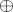is the exclusive-or operation.

### Connections and variations.

The BRG code can be used in solving the Chinese rings puzzle and the towers of Hanoi puzzle, both of which predate Gray's patent [a1]. In practice, variants of the BRG code are often required. Uniform Gray codes refer to those that have, at least approximately, the same number of bit changes occurring in each position. A monotone Gray code has the property that all strings withones appear before any with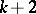ones [a6].

## Combinatorial Gray codes.

The Gray code concept has been extended from subsets to many other types of combinatorial objects. The concept of a combinatorial Gray code is not precisely defined, but the two crucial ideas are that each object is listed exactly once and successive objects differ in some small prescribed way. Combinatorial Gray codes are useful because they often lead to efficient algorithms for generating (i.e., listing) the objects. Some of the more important combinatorial Gray codes are as follows.

### Combinations, partitions, permutations, and other objects.

Represent combinations (cf. Combination) by their characteristic vectors; i.e., the-combinations of an-set are represented by those strings inthat have exactlyones. For combinations, a rule analogous to (a1) may be given, for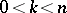. The result is a Gray code in which each string differs from its predecessor by transposition of two bits:This list gives rise to what is known as a revolving door algorithm; one element leaves the set and another element enters the set [a2].

For permutations (cf. Permutation) a Gray code is known in which successive permutations differ only by transposition of two adjacent elements. It is a recursive construction in whichsweeps back and forth through the list of permutations of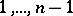. This Gray code is usually attributed to S.M. Johnson and H.F. Trotter [a3], but was known much earlier by experts in campanology (which is the study of bells and bell ringing).

A Gray code is known for the linear extensions of partially ordered sets in which each extension differs by one or two transpositions of adjacent poset elements [a4].

For set partitions, Gray codes are known in which successive partitions differ only by an element moving from some block to some other block. For numerical partitions, a Gray code is known in which successive partitions differ by one part increasing by one and another part decreasing by one [a5].

Many combinatorial Gray codes can be viewed as Hamilton cycles in vertex-transitive graphs, particularly Cayley graphs (cf. also Graph; Graph circuit) [a7]. The Gray code for permutations gives a Hamilton cycle on a Cayley graph known as the permutohedron.

How to Cite This Entry:
Gray code. Encyclopedia of Mathematics. URL: http://encyclopediaofmath.org/index.php?title=Gray_code&oldid=18289
This article was adapted from an original article by F. Ruskey (originator), which appeared in Encyclopedia of Mathematics - ISBN 1402006098. See original article